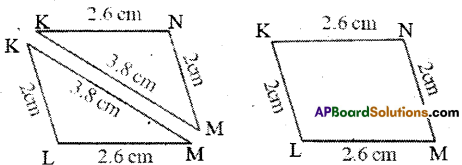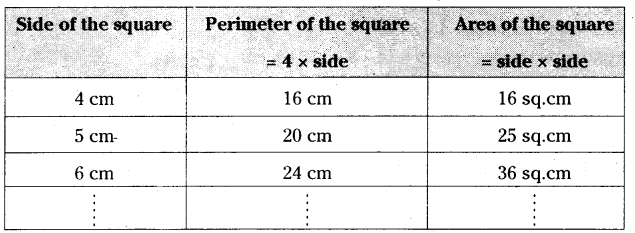# AP Board 6th Class Maths Solutions Chapter 11 Perimeter and Area InText Questions

AP State Syllabus AP Board 6th Class Maths Solutions Chapter 11 Perimeter and Area InText Questions and Answers.

## AP State Syllabus 6th Class Maths Solutions 11th Lesson Perimeter and Area InText QuestionsQuestion 1.
Find the perimeters of the given figures.In the above figures (I) and (ii), find the perimeter of ΔKLM, ΔKMN and □ KLMN.
(a) Compare the perimeters of ΔKLM and □ KLMN
Compare the perimeters of ΔKMN and □ KLMN.
What can you say? –
Solution:
i) In ΔKLM, given KL = 2cm; LM = 2.6 cm; MK = 3.8 cm
Perimeter of ΔKLM = KL + LM + MK
= 2 + 2.6 + 3.8
= 8.4 cmIn ΔKMN, given KM = 3.8 cm; MN = 2cm; KN = 2.6 cm
Perimeter of ΔKMN = KM + MN + KN
= 3.8 + 2 + 2.6 = 8.4 cm

ii) In □ KLMN, given KL = 2cm, LM = 2.6cm, MN = 2cm and KN = 2.6 cm
Perimeter of □ KLMN = KL + LM + MN + KN = 2 + 2.6 + 2 + 2.6 = 9.2 cm

a) By comparing the perimeter of ΔKLM and □ KLMN
8.4 cm < 9.2 cm
∴ Perimeter of ΔKLM < Perimeter of □ KLMN
By comparing the perimeter of ΔKMN and □ KLMN
8.4 cm < 9.2 cm
∴ Perimeter of ΔKMN < Perimeter of □ KLMN.
By comparing the perimeters of triangle with quadrilateral ‘
Perimeter of the triangle is always less than the perimeter of quadrilateral.

Let’s Explore (Pg.No. 150)

Question 1.
If the radius of a circle is doubled, then, what is the change in its circumference?
Solution:
If the radius is r, then its circumference of the circle is 2πr.
If radius is doubled means r = 2r (new)
Write r = 2r in 2πr.
Then, the circumference = 2π x 2r
= 4πr = 2.27πr
Circumference is doubled.
So, if the radius of a circle is doubled, then the circumference is also doubled.

Question 2.
If the radius is halved, then, what is the change in its circumference?
Solution:
If the radius is r, then its circumference of the circle is 2πr.
If radius is halved means r = $$\frac{\mathbf{r}}{2}$$ (new)
Write r = $$\frac{\mathbf{r}}{2}$$ in 2πr.
Then, the circumference = 2π x $$\frac{r}{2}=\frac{1}{2}$$. 2πr
Circumference is halved.
So if the radius of a circle is halved, then the circumference is also halved.Check Your Progress (Page No. 151)

Question 1.
Find the area of a square with side 16 cm.
Solution:
Given side of the square (s) = 16 cm
Area of the square A = s x s = 16 x 16 = 256 sq.cm

Question 2.
Length and breadth of a rectangle are 16 cm and 12 cm respectively. Find its area.
Solution:
Given length of the rectangle l = 16 cm
breadth of the rectangle b = 12 cm
Area of the rectangle A = l x b = 16 x 12 = 192 sq.cm

Let’s Think (Page No. 151)

Question 1.
Find the perimeter and area of a square of side 4 cm. Are these same? Give some examples to support your answer.
Solution:From the above table,
If side is 4 cm, then only its perimeter and areas are equal.
In other cases perimeter and areas are not same.